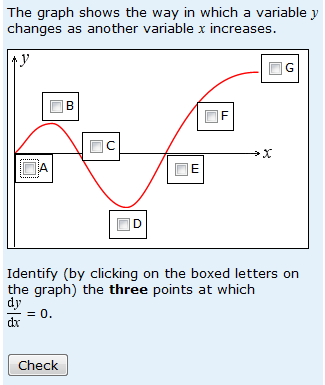# Function or derivative?

The common student error in the question below is somewhat predictable – but I’m not sure why the students make the error that they do.Whilst 60.4% of responses are entirely correct, 21.7% select the three options that are actually the places where the function rather than its derivative is zero. In the existing version of the Maths for Science End of Module Assignment, this question is assessing the contents of a chapter that some students choose not to study – so it is more than usually  likely that a certain percentage of students are guessing, further encouraged by the fact that this is a multiple choice question.

So I’m not sure we can draw many conclusions from the errors that students make on this occasion. But if students really think that dy/dx is zero at the points where the graph crosses the horizontal axis, then they are mistaking the function and its gradient. This is a pretty basic mistake – perhaps a bit like mistaking x to the power of 3 with x times 3. That might be rather interesting.

This entry was posted in derivative, gradient, mathematical misunderstandings and tagged , , . Bookmark the permalink.